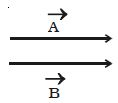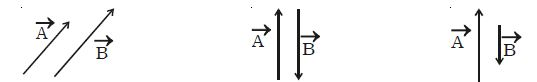Physics

# Different types of Vectors

Here describe different types of Vectors:

(i) Equal vectors

Two vectors are said to be equal if they have the same magnitude and same direction, wherever be their initial positions. In Figure, the vectors Ǡ and Ḃ have the same magnitude and direction. Therefore Ǡ and Ḃ are equal vectors.(ii) Like vectors: Two vectors are said to be like vectors if they have the same direction but different magnitudes as shown in Figure.Like vectors                         Opposite vectors                  Unlike vectors

(iii) Opposite vectors: The vectors of same magnitude but opposite in direction, are called opposite vectors.

(iv) Unlike vectors: The vectors of different magnitude acting in opposite directions are called unlike vectors. In Figure the vectors Ǡ and Ḃ are unlike vectors.

(v) Unit vectors: A vector having unit magnitude is called a unit vector. It is also defined as a vector divided by its own magnitude. A unit vector in the A direction of a vector Ǡ is written as Ǟ and is read as ‘A cap’ or ‘A caret’ or A hat’.